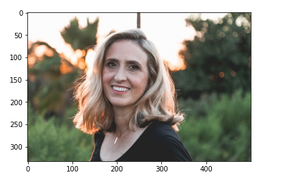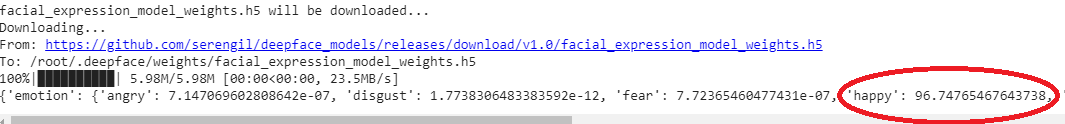# Facial expression detection using Deepface module in Python

• Difficulty Level : Expert
• Last Updated : 28 Jul, 2021

In this article, we are going to detect the facial expression of an already existing image using OpenCV, Deepface, and matplotlib modules in python.

## Module Needed

• OpenCV: OpenCV is an open-source library in python which is used for computer vision, machine learning, and image processing.
• Matplotlib: Matplotlib is a comprehensive library for creating static, animated, and interactive visualizations in Python.
• Deepface: Deepface was built by an artificial intelligence researchers group at Facebook. It is a framework in python for facial recognition and attributes analysis. Deepface’s core library components are used in Keras and TensorFlow.
`pip install deepface`

This is the most basic expression detection technique and there are several ways in which we can detect facial expression.

## Stepwise Implementation

Step 1: Importing the required module.

## Python3

 `#import the required modules ` `import` `cv2 ` `import` `matplotlib.pyplot as plt ` `from` `deepface ``import` `DeepFace`

Step 2: Copy the path of the picture of which expression detection is to be done, read the image using “imread()” method in cv2 providing the path within the bracket. imread() reads the image from the file and stores it in an array. Then use imshow() method of matplotlib. imshow() method converts data into image. Now plot the image using show method in order to ensure that the image has been correctly imported.

## Python3

 `# read image ` `img ``=` `cv2.imread(``'img1.jpg'``) ` ` `  `# call imshow() using plt object ` `plt.imshow(img[:, :, : : ``-``1``]) ` ` `  `# display that image ` `plt.show() `

Output:Output image

Step 3: Create a result variable that will store the result. Use Deepface analyze() method, Deepface analyze() method contains strong facial attribute analysis features such as age, gender, facial expressions. Facial expressions include anger, fear, neutral, sad, disgust, happy, and surprise. Print the result. The result shows the facial expressions percentage of the person.

## Python3

 `# storing the result ` `result ``=` `DeepFace.analyze(img, ` `                          ``actions ``=` `[``'emotion'``]) ` ` `  `# print result ` `print``(result)`

Output:The result shows that person is 96% happy.

Below is the complete implementation:

## Python3

 `# import the required modules ` `import` `cv2 ` `import` `matplotlib.pyplot as plt ` `from` `deepface ``import` `DeepFace ` ` `  `# read image ` `img ``=` `cv2.imread(``'img.jpg'``) ` ` `  `# call imshow() using plt object ` `plt.imshow(img[:,:,::``-``1``]) ` ` `  `# display that image ` `plt.show() ` ` `  `# storing the result ` `result ``=` `DeepFace.analyze(img,actions``=``[``'emotion'``]) ` ` `  `# print result ` `print``(result) `

Output:My Personal Notes arrow_drop_up
Recommended Articles
Page :